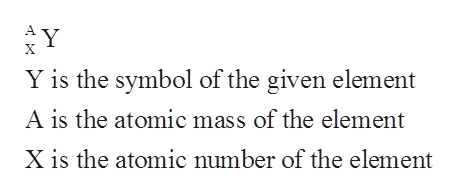# in the equation 228/90Th -------> 228/91Pa + x which particle is represented by X?

Question
16 views

in the equation 228/90Th -------> 228/91Pa + x which particle is represented by X?

check_circle

Step 1

The term atomic number is used to describe the total number of protons which are present in the nucleus of the given atom.

Step 2

The mass number of a given atom is used to describe the total number of protons and neutrons which are present in the nucleus of the atom.

Step 3

The nuclear symbol of a given element includes three main things which are the atomic symbol o...help_outlineImage TranscriptioncloseY A Y is the symbol of the given element A is the atomic mass of the element X is the atomic number of the element fullscreen

### Want to see the full answer?

See Solution

#### Want to see this answer and more?

Solutions are written by subject experts who are available 24/7. Questions are typically answered within 1 hour.*

See Solution
*Response times may vary by subject and question.
Tagged in